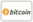Обложка Series Approximation in the Applied Sciences Problems
Название книги:

# Series Approximation in the Applied Sciences Problems

Scholars' Press (2014-11-25 )имеющий право на ваучер
ISBN-13:

### 978-3-639-70985-8

ISBN-10:
3639709853
EAN:
9783639709858
Язык Книги:
Английский
Краткое описание:
The aim of this book is to present new series approximation methods for solving linear and nonlinear differential equations which arising in applied Sciences. These procedures applied on fluid dynamics problems, population model equations, oscillator problems, fractional order equation, batch reactor equation and the Riccati's differential equations. It is interesting to note that these methods give the analytic and numerical results.
Издательский Дом:
Scholars' Press
Веб-сайт:
http://www.scholars-press.com
By (author) :
Najeeb Alam Khan
Количество страниц:
196
Опубликовано:
2014-11-25
Акции:
В наличии
Категория:
Математика
Цена:
79.90 €
Ключевые слова:
fractional calculus, Nonlinear Sciences, series solution### Рассылка